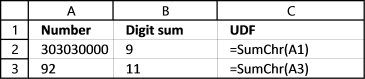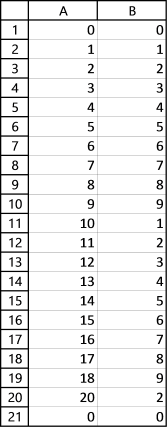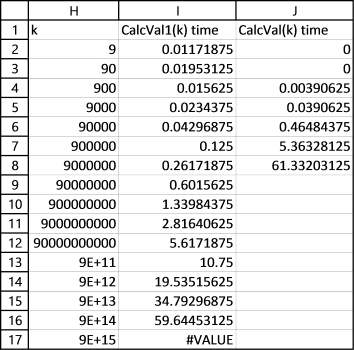Author: Oscar Cronquist Article last updated on January 29, 2018

I got a question a while ago about permutations, in essence how to find every permutation between 0 and 9 000 000 000, if the sum of all digits in a number is smaller or equal to 9.

You can read about the difference between combinations and permutations here: Return all combinations but in short, the order is important for permutations and not important for combinations.

This number 303 030 000 is equal to 9 if you sum every digit, 3+0+3+0+3+0+0+0+0 = 9. 92 is above 9, 9+2 = 11 and is not a number we are looking for.

This user defined function sums all digits in a number.

```Function SumChr(nmbr As Long)
Dim sm As Long
For i = 1 To Len(CStr(nmbr))
sm = sm + Val(Mid(nmbr, i, 1))
Next i
SumChr = sm
End Function
```

Here is a picture of the SumChr function in use.### Easy but slow UDF

It is easy to iterate through every number between 0 and for example 10 000 000 and check if the digit sum is smaller or equal to 9. The following udf does that:

```Function CalcVal(k As Double)
Dim i As Long, j() As Double, a As Double
ReDim j(0 To 60000, 1)
a = Timer
l = 0
For i = 0 To k
m = SumChr(i)
If m = 9 Then
j(l, 0) = i
j(l, 1) = m
l = l + 1
End If
Next i
j(0, 1) = Timer - a
CalcVal = j()
End Function
```

The picture below shows all numbers between 0 and 20 where the digit sum is smaller or equal to 9, the CalcVal function above made this list. Number 19 is missing, 1+9 = 10. 10 is bigger than 9. Column B contains the digit sum, except cell B1. It has the time it took to calculate the list. In this case it took almost no time at all and excel rounded it to 0 (zero).### Much faster UDF

The problem is if you try to do the same with a really large range of numbers, it will take a very long time to calculate for a desktop pc. This udf shortens that time considerably.

```Function CalcVal1(k As Single)
Dim i As Single, j() As Double, a As Double
Dim l As Single, m As Single
a = Timer
ReDim j(0 To 2000000, 1)
i = -1: m = 0
Do Until k &lt;= i
If SumChr(i) = 9 Then
p = Len(CStr(i))
For l = Len(CStr(i)) To 1 Step -1
If Mid(i, l, 1) &lt;&gt; 0 And Mid(i, l, 1) &lt;&gt; 9 Then
i = Val(ReplaceChrInStr(i, l, 0))
i = Val(ReplaceChrInStr(i, l - 1, Mid(i, l - 1, 1) + 1))
Exit For
ElseIf Mid(i, l, 1) = 9 Then
i = i + Application.WorksheetFunction.Power(10, Len(CStr(i)) - 1)
Exit For
End If
Next l
Else
i = i + 1
End If
If i &lt;= k Then
j(m, 0) = i
Else
j(m, 0) = ""
End If
j(m, 1) = SumChr(i)
m = m + 1
Loop
j(0, 1) = Timer - a
CalcVal1 = j
End Function
```

The UDF basically examines each digit in a number and if the sum is 9 these things happens:

• If digit is not equal to 9 and zero, make that digit a 0 and add 1 to the next digit.
• If digit is equal to 9, add 1,10, 100... depending on the position (n) the digit has in the number. Example, digit 9 in number 90 is the second digit counting from right to left, n=2. 10^(2-1) is 10. 90+10 = 100

If the digit sum is not equal to 9 the udf adds 1 to the current number and the loop continues until the entire range has been created.

### Comparing custom functions

Now lets compare the run times of the two udfs CalcVal and CalcVal1.For smaller ranges like 0 - 9000 CalcVal is faster, somewhere around perhaps 5000 CalcVal1 seems to take the lead.

I am using an old laptop with a dual core intel i3 cpu, I am getting 29% utilization. I don't know why, it seems that only one core is utilized by excel. In any case, the numbers indicate that the CalcVal1 function is much faster.